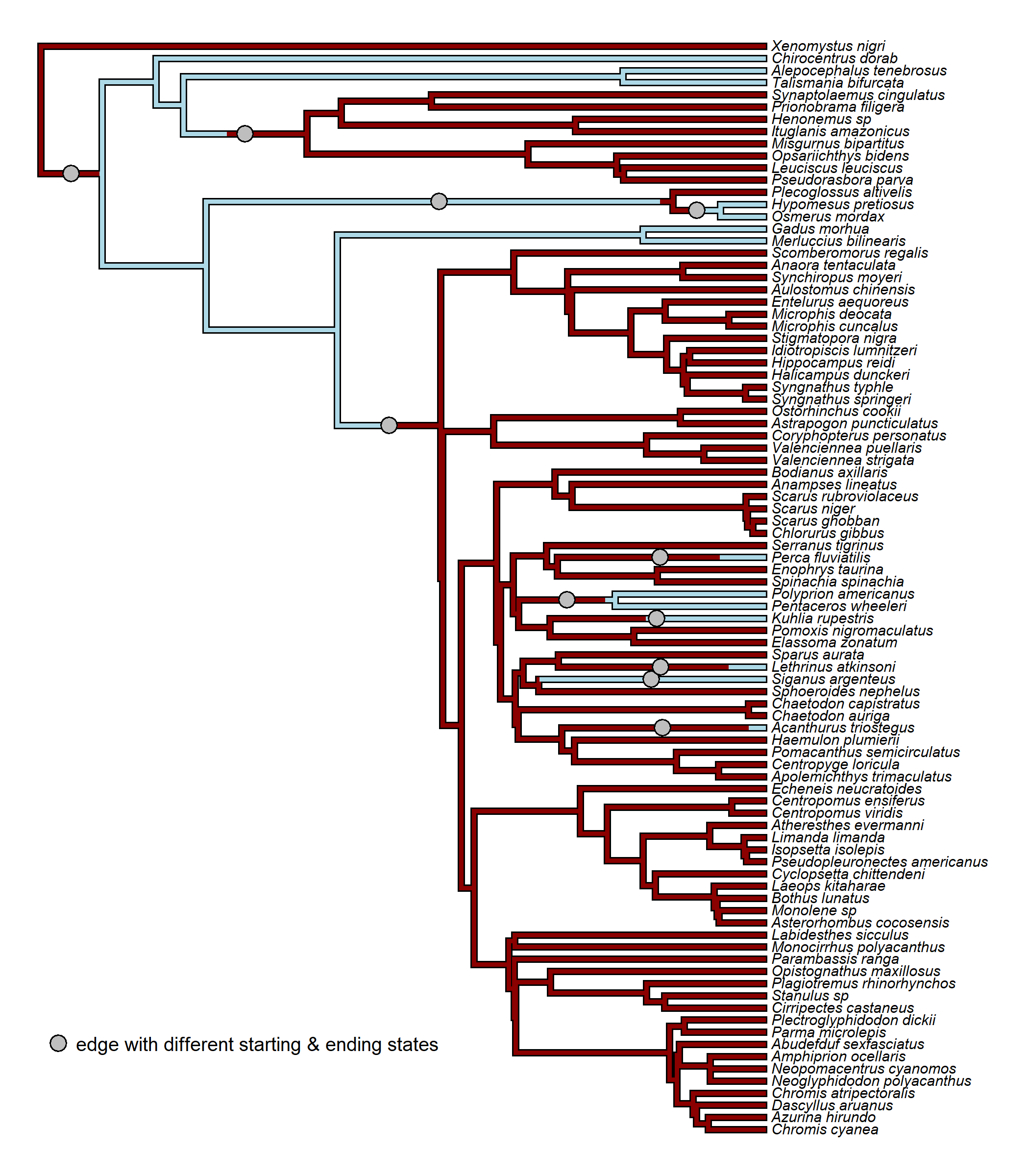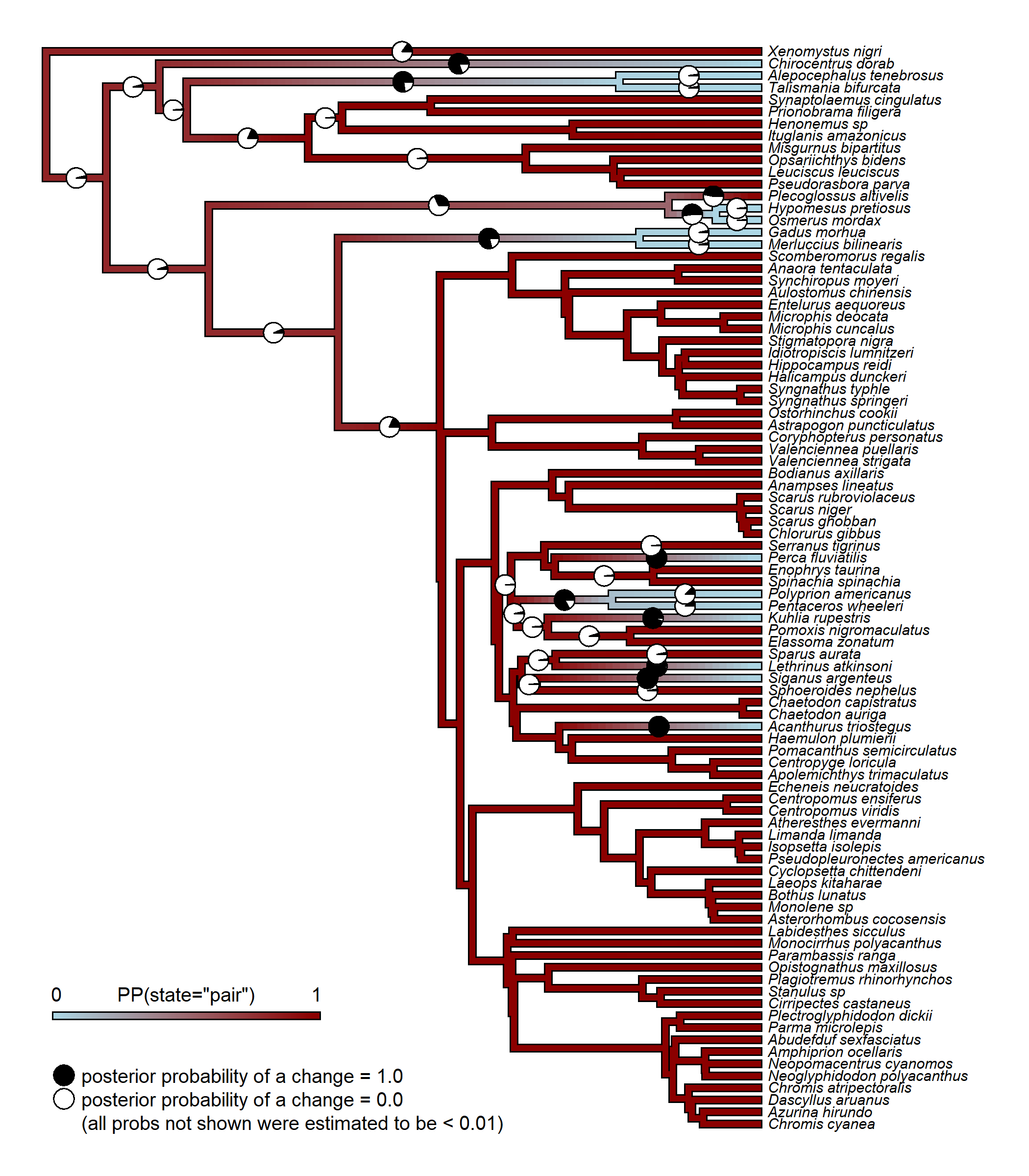## Sunday, October 30, 2022

### Computing and graphing the probabilities of "changes" (different starting & ending states) for each edge of the tree from stochastic mapping

Today, a phytools user wrote:

“I'd like to summarize character reconstructions (using… `make.simmap`) by branch. Specifically I'd like to determine the most likely branch(es) of change in a binary character and then sum these over many characters, all on the same tree. I see several summary functions in phytools but they don't seem to focus on branches, more on state changes.”

phytools has an existing function, `markChanges`, that can be used to tabulate (and, in fact, graph) all the reconstructed character state changes from stochastic mapping on the tree.

This value will include instances in which the character changes state, say, from 0 to 1 and then back again. What I think the user really wants, however, is a set of posterior probabilities that each edge started in one state and ended in the other.

We can definitely compute these probabilities though! Since (to my surprise) I can't recall demonstrating this before, I'll just show it here!

To follow along exactly, I strongly recommend updating phytools from GitHub using devtools, which should give you a version of phytools that matches or exceeds the one reported below:

``````library(phytools)
packageVersion("phytools")
``````
``````##  '1.2.8'
``````

First, I'll assume the character is binary, as indicated by the phytools user (above), and compute a simple probability, for each edge, that (accross our set of maps) a binary trait started each edge in a different state from the one it started it!

In this example, I'll use a tree & dataset from Benun Sutton & Wilson (2019), that I also analyzed in my recent book with Luke Harmon.

``````bonyfish.tree<-read.tree(file="http://www.phytools.org/Rbook/7/bonyfish.tre")
bonyfish.tree
``````
``````##
## Phylogenetic tree with 90 tips and 89 internal nodes.
##
## Tip labels:
##   Xenomystus_nigri, Chirocentrus_dorab, Talismania_bifurcata, Alepocephalus_tenebrosus, Misgurnus_bipartitus, Opsariichthys_bidens, ...
##
## Rooted; includes branch lengths.
``````
``````bonyfish.tree<-ladderize(bonyfish.tree)
row.names=1,stringsAsFactors=TRUE)
``````
``````##                          spawning_mode paternal_care
## Xenomystus_nigri                  pair          male
## Chirocentrus_dorab               group          none
## Talismania_bifurcata             group          none
## Alepocephalus_tenebrosus         group          none
## Misgurnus_bipartitus              pair          none
## Opsariichthys_bidens              pair          none
``````

Our dataset contains two binary variables: spawning mode & the presence or absence of paternal care. We're going to use spawning mode, which can be “group” or “pair”.

Let's start by pulling that out.

``````spawning_mode<-setNames(bonyfish.data\$spawning_mode,
rownames(bonyfish.data))
``````

Now let's pick a model for stochastic mapping.

For a binary character, there are really four basic models: equal-rates, different rates, and the two contrasting irreversible models. Let's fit each of them, but then, for fun, use our model-averaged Q matrix for stochastic mapping.

``````models<-list(ER="ER",ARD="ARD",
Irr1=matrix(c(0,1,0,0),2,2),
Irr2=matrix(c(0,0,1,0),2,2))
fitER<-fitMk(bonyfish.tree,spawning_mode,model="ER",
pi="fitzjohn")
fitARD<-fitMk(bonyfish.tree,spawning_mode,model="ARD",
pi="fitzjohn")
fitIrr1<-fitMk(bonyfish.tree,spawning_mode,
model=matrix(c(0,1,0,0),2,2),pi="fitzjohn")
fitIrr2<-fitMk(bonyfish.tree,spawning_mode,
model=matrix(c(0,0,1,0),2,2),pi="fitzjohn")
w<-anova(fitER,fitARD,fitIrr1,fitIrr2)\$weight
``````
``````##            log(L) d.f.      AIC    weight
## fitER   -29.51819    1 61.03638 0.2955508
## fitARD  -29.23851    2 62.47702 0.1438138
## fitIrr1 -29.25299    1 60.50598 0.3853082
## fitIrr2 -30.04038    1 62.08076 0.1753271
``````
``````Q<-lapply(list(fitER,fitARD,fitIrr1,fitIrr2),as.Qmatrix)
averaged.Q<-Reduce("+",mapply("*",Q,w,SIMPLIFY=FALSE))
mtrees<-make.simmap(bonyfish.tree,spawning_mode,Q=averaged.Q,
pi="fitzjohn",nsim=1000)
``````
``````## make.simmap is sampling character histories conditioned on
## the transition matrix
##
## Q =
##              group         pair
## group -0.001976438  0.001976438
## pair   0.001551502 -0.001551502
## (specified by the user);
## and (mean) root node prior probabilities
## pi =
##     group      pair
## 0.2923999 0.7076001
``````
``````## Done.
``````
``````mtrees
``````
``````## 1000 phylogenetic trees with mapped discrete characters
``````

Now, we're going to compute the frequency, from 1,000 stochastic maps, with which the ending state of each edge was different from the starting state of each edge.

To that, I'll just make a simple function (`foo`) that when I apply it to a specific tree works as follows.

``````foo<-function(x) if(names(x)!=names(x)[length(x)]) 1 else 0
mm<-sapply(mtrees[]\$maps,foo)
mm
``````
``````##    1 0 0 1 0 0 0 0 0 0 0 0 0 0 0 0 0 0 0 0 0 0 0 0 0 0 0 0 0 0 0 0 0 0 0 0
##   0 0 0 0 0 0 0 0 0 0 0 0 0 0 0 0 0 0 0 0 0 0 0 0 0 0 0 0 0 0 0 0 0 0 0 0
##   0 0 0 1 0 0 0 0 0 0 1 0 1 0 0 0 0 0 0 0 1 1 0 0 0 0 0 0 0 1 0 0 0 0 0 0
##  0 0 0 0 0 0 0 0 0 0 0 0 0 0 0 0 0 0 0 0 0 0 0 0 0 0 0 0 0 0 0 0 0 0 0 0
##  0 0 0 0 0 0 0 1 1 0 0 0 0 0 1 0 0 0 0 0 0 0 0 0 0 0 0 0 0 0 0 0 0 0
``````
``````## mark all the edges that satisfy criterion
cols<-setNames(c("lightblue","darkred"),
levels(spawning_mode))
plot(mtrees[],cols,fsize=0.6,ftype="i",lwd=3,outline=TRUE)
edgelabels(edge=which(mm==1),pch=21,cex=1.5,bg="grey")
legend(x=0,y=10,"edge with different starting & ending states",
cex=0.8,pch=21,pt.cex=1.5,pt.bg="grey",bty="n")
``````If we look carefully, we should see that we've correctly identified every edge in which the starting and the ending states differ - right?

Now let's move on to compiling these across all of the trees in our set.

``````pp<-sapply(mtrees,function(x) sapply(x\$maps,foo))
dim(pp)
``````
``````##   178 1000
``````

This matrix is too big to print, but consists of 178 rows (for all the edges of the tree) by 1,000 columns (for all the trees in our set of stochastic character maps). In each matrix position we have a zero if on the *i*th edge of the *j*th tree the ending state & the starting state were not the same.

Now it's easy to estimate the probabilities of changes (defined this way) across all edge – it's simply the row-wise average of `pp`!

``````probs<-apply(pp,1,mean)
probs
``````
``````##    0.039 0.046 0.058 0.178 0.000 0.000 0.000 0.000 0.000 0.000 0.000 0.000
##   0.000 0.000 0.000 0.000 0.000 0.000 0.000 0.000 0.000 0.000 0.000 0.000
##   0.000 0.000 0.000 0.000 0.000 0.000 0.001 0.001 0.000 0.000 0.001 0.000
##   0.000 0.000 0.000 0.000 0.000 0.000 0.000 0.000 0.000 0.000 0.000 0.000
##   0.000 0.000 0.000 0.000 0.000 0.000 0.000 0.000 0.000 0.000 0.000 0.000
##   0.000 0.000 0.000 0.000 0.001 0.001 0.000 0.004 0.002 0.004 0.000 0.000
##   0.000 0.000 0.004 0.994 0.002 0.000 0.000 0.002 0.013 0.017 0.983 0.023
##   0.973 0.027 0.011 0.031 0.015 0.045 0.009 0.009 0.946 0.836 0.121 0.121
##   0.003 0.003 0.013 0.003 0.003 0.984 0.013 0.000 0.000 0.000 0.000 0.000
##  0.000 0.000 0.000 0.000 0.000 0.000 0.001 0.001 0.000 0.000 0.000 0.000
##  0.002 0.001 0.001 0.000 0.000 0.000 0.000 0.000 0.000 0.000 0.000 0.000
##  0.000 0.000 0.000 0.000 0.000 0.000 0.000 0.000 0.000 0.000 0.000 0.000
##  0.000 0.000 0.000 0.000 0.792 0.030 0.030 0.321 0.523 0.012 0.012 0.465
##  0.037 0.016 0.184 0.018 0.005 0.000 0.000 0.000 0.000 0.005 0.012 0.007
##  0.002 0.002 0.005 0.004 0.004 0.776 0.025 0.025 0.801 0.157
``````

Lastly, let's graph these probabilities onto the tree. I'll overlay them on a `"densityMap"` style visualization so that we can see that we have, in fact, computing the correct values. To avoid a cluttered graph, I'll only show probabilities in excess of 0.01.

``````obj<-densityMap(mtrees,plot=FALSE)
``````
``````## sorry - this might take a while; please be patient
``````
``````obj<-setMap(obj,cols)
plot(obj,fsize=c(0.6,0.9),outline=TRUE,lwd=4,legend=FALSE)
ee<-which(probs>=0.01)
edgelabels(edge=ee,pie=cbind(probs[ee],1-probs[ee]),
cex=0.5,piecol=c("black","white"))
legend(x=0,y=7,c("posterior probability of a change = 1.0",
"posterior probability of a change = 0.0",
"(all probs not shown were estimated to be < 0.01)"),
pch=c(21,21,NA),pt.bg=c("black","white",NA),pt.cex=1.9,
cex=0.8,bty="n")If we wanted to define change in this way, then we could update our prior code to simply ask if `maps`, instead of differing in state across its length, had a length in excess of 1. I.e., define `foo` as `foo<-function(x) if(length(x)>1) 1 else 0` and leave everything else the same.